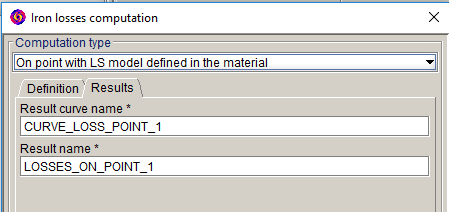# Results of LS iron losses computation on point

## Computation results

The computation of LS iron losses on point gives the following results## Curve

"CURVE_LOSS_POINT_i" curve can be displayed automatically and is stored in the tree in the area Postprocessing > Curve > 2D Curve (I/O Parameter)

It contains three curves:
• volume density of instantaneous losses on the point versus time "DVOL_LOSS_LS_INST"
• Magnetic flux density on point versus time "BMAG1_LS”
• magnetic field on point versus time "HMAG1_LS”

Remark: the last two curves show the hysteresis cycle on the considered point.

## Result

The results are automatically displayed in the data tree in the area Postprocessing > Result > Iron losses Result.

The results include:
• Volume density of average iron losses over a period on the point (in W/m3)
• Volume density of iron losses energy over a period on the point (in J/m3)